NCERT Solutions for Class 8 Maths Chapter 15 Introduction to Graphs Ex 15.3 are part of NCERT Solutions for Class 8 Maths. Here we have given NCERT Solutions for Class 8 Maths Chapter 15 Introduction to Graphs Ex 15.3.

 Board CBSE Textbook NCERT Class Class 8 Subject Maths Chapter Chapter 15 Chapter Name Introduction to Graphs Exercise Ex 15.3 Number of Questions Solved 2 Category NCERT Solutions

## NCERT Solutions for Class 8 Maths Chapter 15 Introduction to Graphs Ex 15.3

Question 1.
Draw the graphs for the following tables of values, with suitable scales on the axes.
(a) Cost of apples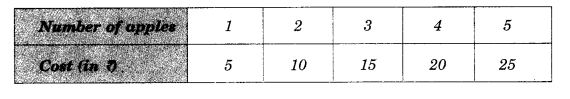(b) Distance travelled by a car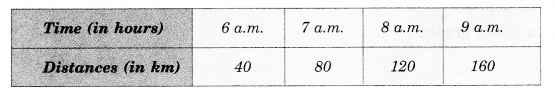(i) How much distance the car cover during the period 7.30 a.m. to 8 a.m.?
(ii) What was the time when the car had covered a distance of 100 km since it starts?

(c) Interest on deposits for a year.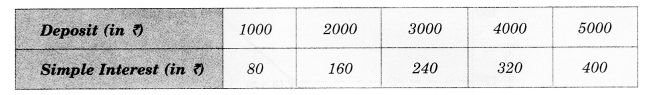(i) Does the graph pass through the origin?
(ii) Use the graph to find the interest on? 2500 for a year.
(iii) To get an interest of ? 280 per year, how much money should be deposited?
Solution.
(a)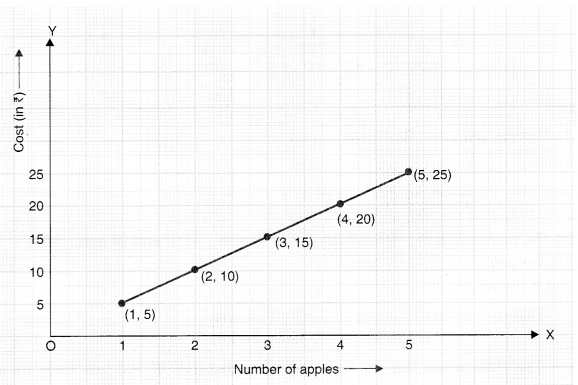Scale:
On horizontal axis:         2 units = 1 apple
On vertical axis:              1 unit = ₹ 5
Mark number of apples on the horizontal axis.
Mark cost (in ?) on the vertical axis.
Plot the points: (1, 5), (2, 10), (3, 15), (4, 20) and (5, 25)
Join the points.
We get a linear graph.

(b)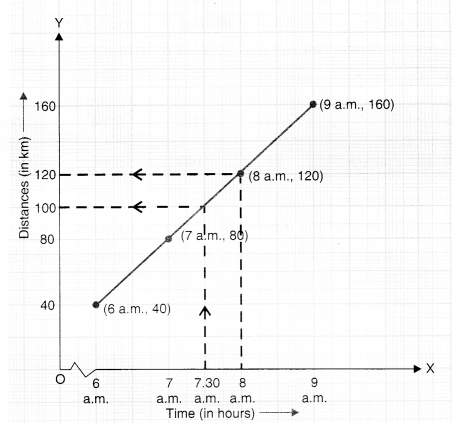Scale:
On horizontal axis:      2 units = 1 hour
On vertical axis:           2 units = 40 km
Mark time (in hours) on the horizontal axis.
Mark distances (in km) on the vertical axis.
Plot the points (6 a.m., 40), (7 a.m., 80) (8 a.m., 120) and (9 a.m., 160).
Join the points.
We get a linear graph.
(i) Distance covered during 7.30 a.m. to 8 a.m.
= 120 km – 100 km = 20 km
(ii) The time when the car had covered a distance of 100 km since its start was 7.30 a.m.

(c)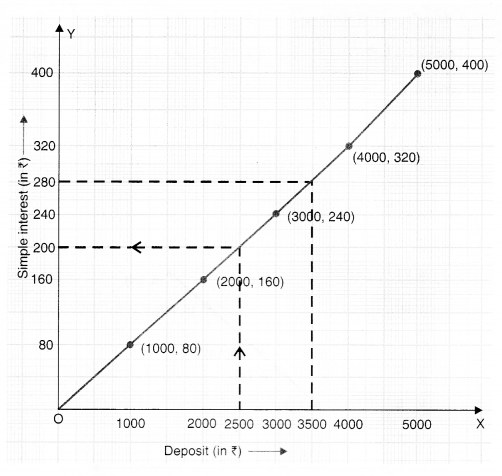Scale:
On horizontal axis:       2 units = ₹ 1000
On vertical axis:            2 units = ₹ 80
Mark deposit (in ₹ on the horizontal axis.
Mark simple interest (in ₹) on the vertical axis.
Plot the points (1000, 80), (2000, 160), (3000, 240) (4000, 320) and (5000, 400).
Join the points.
We get a linear graph.
(i) Yes! The graph passes through the origin.
(ii) Interest on ₹ 2500 for a year = ₹ 200
(iii) To get an interest of ₹ 280 per year, ₹ 3500 should be deposited.

Question 2.
Draw a graph for the following:
(i)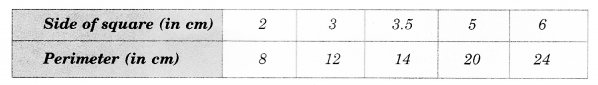Is it a linear graph?
(ii)Is it a linear graph?
Solution.
(i)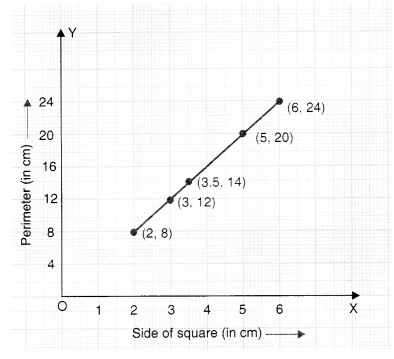Scale:
On horizontal axis:     1 unit = 1 cm
On vertical axis:          1 unit = 4 cm
Mark side of the square (in cm) on the horizontal axis.
Mark perimeter (in cm) on the vertical axis.
Plot the points (2, 8), (3, 12), (3.5. 14) (5, 20) and (6, 24).
Join the points.
Yes ; it is a linear graph.

(ii)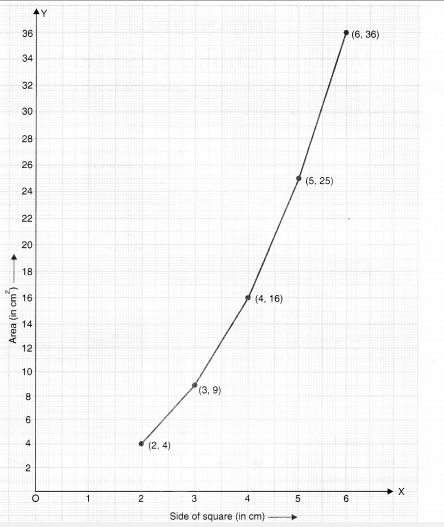• Scale:
On horizontal axis: 2 units = 2 cm
On vertical axis: 1 unit = 2 cm
Mark side of the square (in cm) on the horizontal axis.
Mark area (in cm ) on the vertical axis.
Plot the points (2, 4) (3, 9), (4, 16), (5, 25) and (6, 36).
Join the points.
The graph we get is not linear.

We hope the NCERT Solutions for Class 8 Maths Chapter 15 Introduction to Graphs Ex 15.3 help you. If you have any query regarding NCERT Solutions for Class 8 Maths Chapter 15 Introduction to Graphs Ex 15.3, drop a comment below and we will get back to you at the earliest.